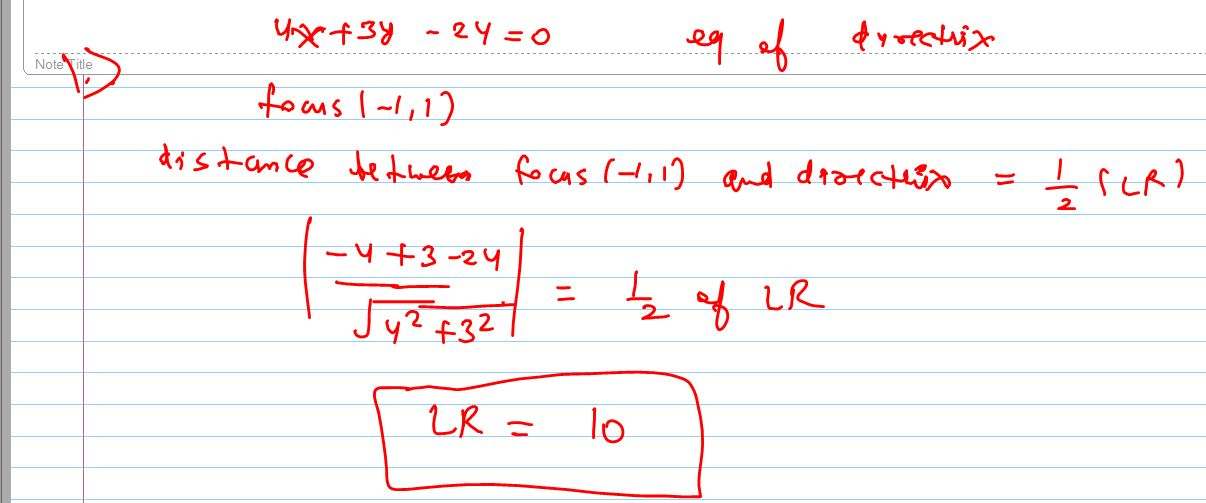# paraboIa 1]   Iength of Iatus rectum whose focus is {-1 1}& directrix 4x+3y-24=0? 2]  2x+y+k=0[k is a constant]is a normaI to y^2=-8x ,then k=? 3]  sIope of commom tangent to paraboIa y^2=16x & x^2=2y is? 4]  a straight Iine G whvse sIope is m, passes through pt {2 0} and intersects y^2-4x+4=0 in distinct pts, hence m Iies in the intervaI? 5]  the extremities of Iatus rectum of a paraboIa are {3 -2} & {3 0}, if the foot of directrix is in the 4th quadrant then the eqt of directrix can be?Deepak Kumar Shringi